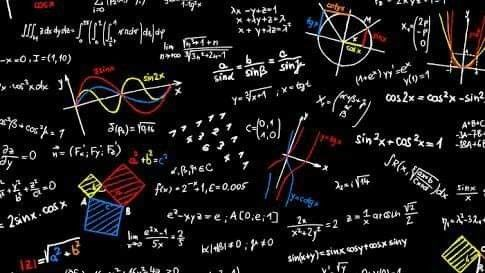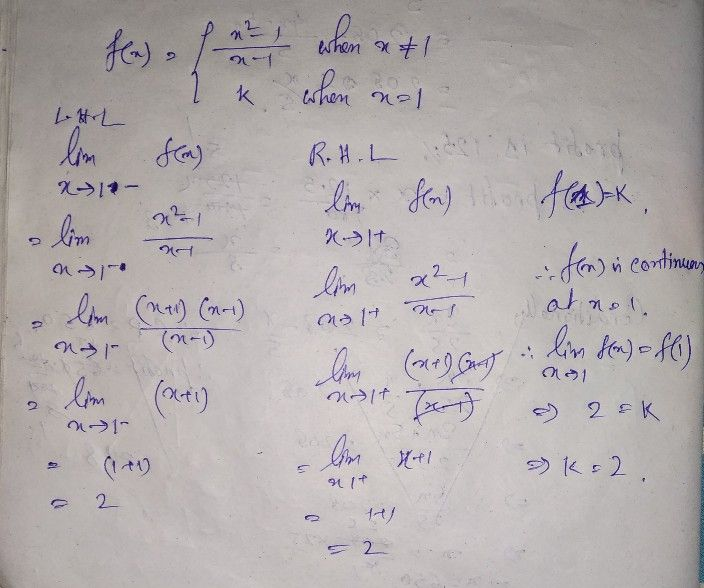Symbol
Problemwhen $x≠1$ If the function $f\left(x\right)$ $= \begin{cases} \dfrac {x^{2}-1} {x-1} \\ k \end{cases}$ when $x=1$ is given to be continuous at $x=1,$ then the value of $k$ is
Other
SolutionQanda teacher - StudyPoint